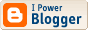How to Crash InDesign

Use the script in that last post from yesterday on a table of 10 or more rows!

Why does it crash? Because of a stupid error that is hard to see. The problem is that the start and end values I'm calculating are strings, not numbers, so the internal loop compares (in the case I was working with) 2 with 11 and concludes that 2 is greater than 11 (because as strings, that's true) and so the loop doesn't execute. This results in the script concluding that each of the columns should have a width of Infinity.

Believe it or not, this works, causing the table to become overset (there's a surprise). You can even undo to get back to where you were. But if you don't and you try to work with the document it will crash and then it is irretrievably corrupted.

Here's a corrected version of the script. You'll notice I changed the names of the arguments to getCell because I thought that might have something to do with the problem until I realized what was really going on.
//DESCRIPTION: Table Space Equalizer

/*
Expects a multi-column selection within a table. Adjusts widths of selected
columns so that space after the longest entry in each column is equalized and
the overall width of the table is unchanged.
*/

if ((app.documents.length == 0) || (app.selection.length == 0)) { exit() }

aSel = app.selection;

try {
theCols = {start: Number(aSel.cells.name.split(":")),end: Number(aSel.cells[-1].name.split(":"))};
theRows = {start: Number(aSel.cells.name.split(":")),end: Number(aSel.cells[-1].name.split(":"))};
theTable = aSel.parent;
} catch (e) {
alert("Selection needs to be in a table, and rectangular"), exit();
}

// For each column, calculate the min spare space:
minSpaces = new Array();
for (c = theCols.start; theCols.end >= c; c++) {
minSpace = Infinity;
for (r = theRows.start; theRows.end >= r; r++) {
minSpace = Math.min(minSpace, space(getCell(theTable, c, r,)));
}
minSpaces.push(minSpace);
}
totSpace = 0;
for (j = 0; minSpaces.length > j; j++) {
totSpace = totSpace + minSpaces[j]
}
newSpace = totSpace/minSpaces.length;
for (c = theCols.start; theCols.end >= c; c++) {
theTable.columns[c].width = theTable.columns[c].width + newSpace - minSpaces[c - theCols.start];
}

function getCell(table, n, m) {
return table.cells.item(n + ":" + m);
}

function space(theCell) {
// This version assumes that the cell is left aligned and there's only one line
var left = theCell.texts.insertionPoints.horizontalOffset;
theCell.texts.justification = Justification.rightAlign;
// theCell.texts.recompose();
var spare = theCell.texts.insertionPoints.horizontalOffset - left;
theCell.texts.justification = Justification.leftAlign;
return spare;
}